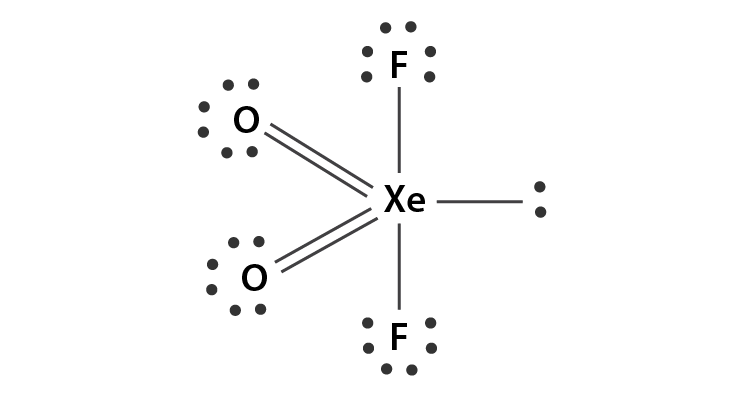# Hybridization of XeO2F2 (Xenon Dioxide Difluoride)

Students can easily determine the hybridization of XeO2F2 by knowing the number of valence electrons and using the basic hybridization formula which is given as – Number of electrons = ½ [V+N-C+A].

Here, V will be the number of valence electrons present in the central atom (xenon).

N will be equal to the number of monovalent (fluorine) atoms bonded to the central atom. C will be the charge of cation and A will be the charge of anion.

We will look at the process in detail below.

 Name of the Molecule Xenon Dioxide Difluoride Molecular Formula XeO2F2 Hybridization Type sp3d Bond Angle 91o 105o and 174o Geometry Trigonal Bipyramidal or See Saw

## What is the Hybridization of XeO2F2?

In Xenon Dioxide Difluoride, xenon will be the central atom which will have 8 valence electrons. The fluorine atom will be the monovalent surrounding atom and the oxygen atom will be the divalent surrounding atom. We will take the eight valence electrons of Xenon and add 2 monovalent fluorine atoms. The whole sum will be divided by 2 at the end.

If we take the values then;

Number of electrons = ½ [8+2-0+0]

= 5

We get 5 as the final number which further suggests sp3d hybridization. In Xenon Dioxide Difluoride, there will be 5 sp3d hybrid orbitals. There are 5 electron pairs around the centre atom where it will contain 4 bond pairs and 1 lone pair.### Important Points To Remember

• The hybridization of Xenon which is the central atom is sp3d.
• The central atom will contain 4 bond pairs and 1 lone pair.
• Xenon basically belongs to the noble gas family. What this means is that it does not have to form any bonds to complete its octet.

## XeO2F2 Molecular Geometry And Bond Angles

XeO2F2 molecular geometry is originally said to be trigonal bipyramidal but due to the presence of lone pair on equatorial position, the actual shape will be see-sawed. The repulsion between bond pair and lone pair of electrons will be more. Here, fluorine will be axial atoms and oxygen will be equatorial atoms. As for the angles, the O–Xe–O angle will be 105.7°, O–Xe–F will be 91.6° and F–Xe–F will be 174.7°.